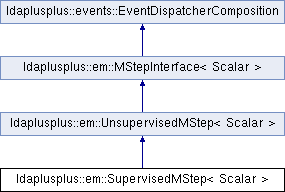LDA++
ldaplusplus::em::SupervisedMStep< Scalar > Class Template Reference

#include <SupervisedMStep.hpp>

Inheritance diagram for ldaplusplus::em::SupervisedMStep< Scalar >:## Public Member Functions

SupervisedMStep (size_t m_step_iterations=10, Scalar m_step_tolerance=1e-2, Scalar regularization_penalty=1e-2)

virtual void m_step (std::shared_ptr< parameters::Parameters > parameters) override

virtual void doc_m_step (const std::shared_ptr< corpus::Document > doc, const std::shared_ptr< parameters::Parameters > v_parameters, std::shared_ptr< parameters::Parameters > m_parameters) overridePublic Member Functions inherited from ldaplusplus::events::EventDispatcherComposition
std::shared_ptr< EventDispatcherInterfaceget_event_dispatcher ()

void set_event_dispatcher (std::shared_ptr< EventDispatcherInterface > dispatcher)

## Detailed Description

### template<typename Scalar> class ldaplusplus::em::SupervisedMStep< Scalar >

SupervisedMStep implements the M step for the categorical supervised LDA.

As in FastSupervisedMStep we delegate the maximization with respect to $$\beta$$ to UnsupervisedMStep and then maximize the the lower bound of the log likelihood with respect to $$\eta$$ using gradient descent.

The difference of SupervisedMStep compared to FastSupervisedMStep is that this class uses the second order taylor approximation (instead of the first) to approximate $$\mathbb{E}_q[\log p(y \mid z, \eta)]$$.

$\mathcal{L}_{\eta} = \sum_{d=1}^D \eta_{y_d}^T \mathbb{E}_q[\bar{z_d}] - \sum_{d=1}^D \log \sum_{\hat{y}=1}^C \exp(\eta_{\hat{y}}^T \mathbb{E}_q[\bar{z_d}]) \left( 1 + \frac{1}{2} \eta_{\hat{y}}^T \mathbb{V}_q[\bar{z_d}] \eta_{\hat{y}} \right)$

This approximation has been used in  but it is slower and requires huge amounts of memory for even moderately large document collections.

 Chong, Wang, David Blei, and Fei-Fei Li. "Simultaneous image classification and annotation." Computer Vision and Pattern Recognition, 2009\. CVPR 2009. IEEE Conference on. IEEE, 2009.

## Constructor & Destructor Documentation

template<typename Scalar >
 ldaplusplus::em::SupervisedMStep< Scalar >::SupervisedMStep ( size_t m_step_iterations = 10, Scalar m_step_tolerance = 1e-2, Scalar regularization_penalty = 1e-2 )
inline
Parameters
 m_step_iterations The maximum number of gradient descent iterations m_step_tolerance The minimum relative improvement between consecutive gradient descent iterations regularization_penalty The L2 penalty for logistic regression

## Member Function Documentation

template<typename Scalar >
 void ldaplusplus::em::SupervisedMStep< Scalar >::doc_m_step ( const std::shared_ptr< corpus::Document > doc, const std::shared_ptr< parameters::Parameters > v_parameters, std::shared_ptr< parameters::Parameters > m_parameters )
overridevirtual

Delegate the collection of some sufficient statistics to UnsupervisedMStep and keep in memory $$\mathbb{E}_q[\bar z_d]$$ and $$\mathbb{V}_q[\bar z_d]$$ for use in m_step().

Parameters
 doc A single document v_parameters The variational parameters used in m-step in order to maximize model parameters m_parameters Model parameters, used as output in case of online methods

Reimplemented from ldaplusplus::em::UnsupervisedMStep< Scalar >.

template<typename Scalar >
 void ldaplusplus::em::SupervisedMStep< Scalar >::m_step ( std::shared_ptr< parameters::Parameters > parameters )
overridevirtual

Maximize the ELBO w.r.t. to $$\beta$$ and $$\eta$$.

Delegate the maximization regarding $$\beta$$ to UnsupervisedMStep and maximize $$\mathcal{L}_{\eta}$$ using gradient descent.

Parameters
 parameters Model parameters (changed by this method)

Reimplemented from ldaplusplus::em::UnsupervisedMStep< Scalar >.

The documentation for this class was generated from the following files: